## 1. Overview

The software oriented libraries contains functionality which runs normally in a controller. In opposite the hardware oriented libraries runs never in a controller, it are helpers for the environment simulation. The software oriented libraries can also be used for hardware (environment) simulation

Software oriented libraries:

• libVishia_Conv: Some conversion routines, data formats, bit presentation etc.

• libVishia_Ctrl: Control FBlocks, for example OrthOsc, T1-FBlocks

• libVishia_CtrlSfn: Control FBlocks as S-Functions, for example PID, OrthOsc

• libVishia_Inspc: The Inspector FBlocks, also TimeSignals.

• libVishia_EvStm: FBlocks as S-Function for events and state machines.

Environment oriented libraries:

• libVishia_Electric: Uses the older SimPowerSystem

## 2. libVishia_Conv

This lib contains some FBlocks for simple conversions. Either they are only a change of representation of the data (signed with (T) = "Type"), or simple calculations (signed with (F) = Function). FBlocks with states (signed with (Q) = "State") are not member of this lib.

Complex representation, vector and scalar

•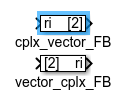(T) cplx_vector_FB, vector_cplx_FB: converts a complex representation in a vector and back. A complex number is well defined in operations, but it can be converted to a simple representation as vector. Last one is necessary for data transfer in an array, for example to assign to a scope together with other scalars and vectors using a mux.

•(F) cplxni_FB: This FBlock changes only the sign of the imagin part. It can be used to change the rotating direction of a complex oscillating value to backward. It is important for the Park transformation, see next.

•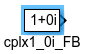(T) cplx1_0i_FB: This is only a value for the complex pointer to (1 + 0i). It is difficult to present with a const block in Simulink, because 0*i is calculated as 0 (scalar).

•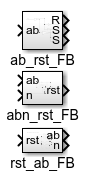(F) ab_rst_FB, abn_rst_FB, rst_ab_FB, : (F) That are three FBlocks for Clarke transformation and back Clarke. The https://en.wikipedia.org/wiki/Alphabeta_transformation transforms a given 3-phase signal in a (usual) symmetrical electrical grid to a complex vector. The 'n' input is the null-system, it is 0 in a symmetrical net. The three values ab (complex, 2 values) and 'n' represents bijective the three phase values 'rst'. It is also in timing. It is not the transformation to a phasor system which only calculates with the fundamental components. All harmonics and negative system components are remain containing. The 3 phase values are given as vector with three components. The ab_rst_FB is a simple form with 3 scalar outputs for a signal without null-system which can be often internally used.

Angle and frequency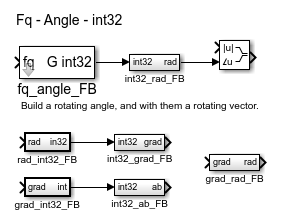This FBlocks work generally with an `int32` representation of the angle. The value `0x7fffffff` presents the angle 179.9999 degree, and `0x80000000` is the 180° or -180°. The advantage of this representation: Clips to pi and -pi are not necessary. The angle is always well defined in range -pi …​ pi. But the more important advantage: Differences of angles are simple calculated. The difference between for example -179° and 179° is of course 2°, without numerical effort. Also a filtering with a T1_FB is possible in the range about 180°. The next advantage: The solution is 32 bit, always constant. The `float` or `single` representation has only 24 bit.

• (Q) fq_angle_FB: This is a FBlock which converts a given frequency to a current rotating angle. The important parameter is the step time, initial given as 'TstepHw'. This FBlock can often be used to build an currently angle for a electric simulation. The FBlock contains a state, it is the current angle itself.

• (F) int32_rad_FB: converts to the angle-32 value to use in float calculations (input for sin etc.or to convert to complex as shown).

• (F) int32_grad_FB: converts to the angle-32 value to use as degree value, usual for display.

• (F) rad_int32_FB: converts from -pi..pi to the 32-bit-angle. If the angle value is outside the range of -pi .. pi this FBlock should calculate the correct angle value modulo the normalized angle range. It is true for Simulink on a PC, it should be true also for the target system. The code generation should be tested (with the designated target system, it is a property of the compiler and machine instruction) whether it is correct.

• (F) grad_int32_FB: converts a degree value, usual from manual inputs, to the int32 representation. The rule for degree values outside -180..179.999 is the same as for rad_int32_FB.

• (F) grad_rad_FB: It contains only a gain FBlock with the correct association between degree and radian.

• (F) int32_ab_FB: This FBlock calculates from given angle-32 to a complex vector. The angle 0 is the value (1 + 0i). Angle = `0x40000000` = 90° or pi/2 is (0 + 1i). This FBlock contains a sin and a cos function call. Be aware, it is some expensive on the target hardware.

Boolean conversions

The boolean type as two values, true = 1 or false = 0. It is like a binary signal in hardware. Unfortunately a breaker in the Simulink’s electric simulation (Sim Power Systems) or some switches in the base FBlocks uses a double value as binary input. But nevertheless the user should use boolean where boolean values are required. With the following FBlocks boolean values can be presented as bit values in integer, as it is familiar in controller programming.

•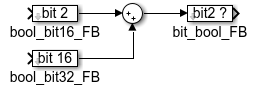(F) bool_bit16_FB_Conv: This FBlock presents a boolean value as bit in an `uint16` value. The bit is given as parameter 0..15 Only one bit can be presented. If more outputs of such FBlocks are ored or added, a bit field can be built to present some booleans, as shown in the graphic.

• (F) bool_bit32_FB_Conv: This FBlock presents a boolean value as bit in an `uint32` value. The bit is given as parameter 0..31 Only one bit can be presented. If more outputs of such FBlocks are ored or added, a bit field can be built to present some booleans, as shown in the graphic.

• (F) bit_bool_FB_Conv: This FBlock is the revers to bool_bit16_FB_Conv and bool_bit32_FB_Conv. The input can use any `int` format, but the bit parameter should match to the input bit width. It checks the numbered bit from 0.. and outputs true if the bit is set in the input value.

•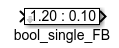(F) bool_single_FB_Conv: This FBlock outputs the dedicated values for true and false. true is represented with the 'hi' value, shown left in the FBlock, false is 'lo' shown right side. This FBlock can be especially used to connect boolean values to a Mux for an scope input. With the value levels more as one boolean signal can be presented in one track.

•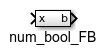(F) num_bool_FB_Conv: It is a simple FBlock to present any numerical value as boolean. As in C language the value 0 is false, not 0 is true. All other checks of numericals should be done with the standard Simulink FBlocks (comparator).

•(F) Fader_FB_Conv: This FBlock has an input value between 0.0 and 1.0 for fading. It presents the 'x0' value on 'y' for the fading input 0.0 and the 'x1' value for fading input 1.0, and any value between that. If the fading value is < 0.0, 0.0 is used. Adequate is with >1.0, using 1.0. It is a simple numerical algorithm with an limitation.